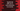# TypeScript add one or more elements to an array# Introduction :

In typescript, we have a couple of different ways to add elements to an array. We can add elements to the start of the array, end of an array or to the middle of an array. In this post, I will show you different ways to do that with examples.

## Method 1: Using push :

push() is used to append elements to the end of an array. pop(), the other opposite method, removes elements from the end. We can use push() to add single or multiple elements to an array. For example :

``````let givenArray = [1,2,3,4,5]
givenArray.push(6)

console.log(givenArray)``````

It will print :

``[ 1, 2, 3, 4, 5, 6 ]``

Similarly, we can use it for adding multiple elements :

``````let givenArray = [1, 2, 3, 4, 5]
givenArray.push(6, 7, 8, 9, 10)

console.log(givenArray)``````

Output :

``[ 1, 2, 3, 4, 5, 6 ]``

This is the easiest option to add items to an array.

## 2. Using unshift() :

Similar to push-pop, unshift and shift is used to add, remove elements from the start of an array. unshift adds one or more elements and shift removes elements. For example :

``````let givenArray = [1, 2, 3, 4, 5]
givenArray.unshift(0)

console.log(givenArray)``````

It prints :

``[ 0, 1, 2, 3, 4, 5 ]``

With multiple values :

``````let givenArray = [1, 2, 3, 4, 5]
givenArray.unshift(-2, -1, 0)

console.log(givenArray)``````

Output :

``[ -2, -1, 0, 1, 2, 3, 4, 5 ]``

## Using index notation :

The index of array elements starts from 0. We can directly modify any element of an array using its index. Similarly, you can also add items to the end of an array using its index. If the array has x number of elements, you can add elements to index x+1, x+2..etc using index. For example :

``````let givenArray = [1, 2, 3, 4, 5]
givenArray = 6

console.log(givenArray)``````

It will print :

``[ 1, 2, 3, 4, 5, 6 ]``

We can add an infinite number of elements using index notation.

## Using concat :

concat() method joins two arrays and returns the new array. It doesn’t modify the original array. For example :

``````let givenArray = [1, 2, 3, 4, 5]
let newArray = givenArray.concat([6, 7, 8, 9, 10])

console.log(newArray)``````

It appends the new array elements to the end of newArray and returns one new array. The above program prints the below output :

``[ 1, 2, 3, 4, 5, 6, 7, 8, 9, 10 ]``

If you want to add the elements to the start :

``````let givenArray = [1, 2, 3, 4, 5]
let newArray = [6, 7, 8, 9, 10].concat(givenArray)

console.log(newArray)``````

Output :

``[ 6, 7, 8, 9, 10, 1, 2, 3, 4, 5 ]``

## Using splice :

splice() method is used to add elements to the middle of an array. The syntax of this method is as below :

``array.splice(start[, count[, item1[, item2[, ...]]]])``

It starts the deletion of array items from index start, count is the number of elements to delete starting from index start and item1, item2, _etc. are items to add to the array starting from _start index. In this example, we are not deleting any elements. So, we will give count as 0.

Add to the start of an array :

``````let arr = [1, 2, 3, 4, 5]

arr.splice(0, 0, 6, 7, 8, 9)

console.log(arr)``````

Output :

``[6, 7, 8, 9, 1, 2, 3, 4, 5]``

Add to the end of an array :

``````let arr = [1, 2, 3, 4, 5]

arr.splice(arr.length, 0, 6, 7, 8, 9)

console.log(arr)``````

Output :

``[1, 2, 3, 4, 5, 6, 7, 8, 9]``

Adding elements to the middle of the array :

``````let arr = [1, 2, 3, 4, 5]

arr.splice(2, 0, -1, -2, -3)

console.log(arr)``````

Output :

``[1, 2, -1, -2, -3, 3,  4,  5]``